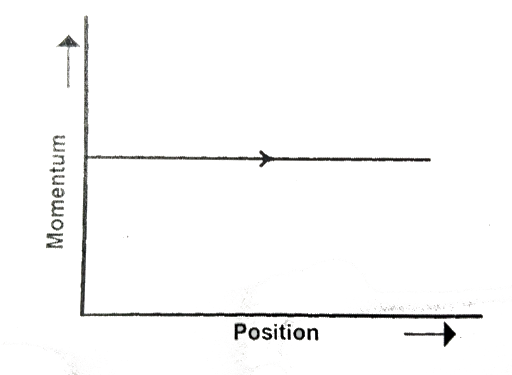Phase space diagrams are useful tools in analyzing all kinds of dy | Filoclass 12

Missing544

Phase space diagrams are useful tools in analyzing all kinds of dynamical problems. They are especially useful is studying the changes in motion as initial position and momentum are changed. Here we consider some simple dynamical systems in one-dimensions. For such systems, phase space is a plane in which position is plotted along horizontal axis and momentum is plotted along vertical axis. The phase space diagram is x(t) vs. p(t) curve in this plane. The arrow on the curve indicates the time flow. For example, the phase space diagram for a particle moving with constant velocity is a straight line as shown in the figure. We use sign convention in which position or momentum upwards (or to right) is positive and downwards (or to left) is negative.The phase space diagram for a ball thrown vertically up from ground is544Connecting you to a tutor in 60 seconds.